# dsp.DynamicFilterVisualizer

Display time-varying magnitude and phase response of digital filters

## Description

The `dsp.DynamicFilterVisualizer` object displays the magnitude response and phase response of time-varying digital filters or time-varying filter coefficients. The input to this object can be a filter coefficients vector or a filter System object™.

Using the dynamic filter visualizer, you can configure the plot settings, find the peak values, enable cursor measurements, and even generate script to recreate the plot with the current settings from the visualizer interface. For details, see Configure Filter Visualizer.

## Creation

### Syntax

``dfv = dsp.DynamicFilterVisualizer``
``dfv = dsp.DynamicFilterVisualizer(nfft)``
``dfv = dsp.DynamicFilterVisualizer(nfft,Fs)``
``dfv = dsp.DynamicFilterVisualizer(nfft,Fs,range)``
``dfv = dsp.DynamicFilterVisualizer(Name=Value)``

### Description

example

````dfv = dsp.DynamicFilterVisualizer` returns a dynamic filter visualizer object, `dfv`, that displays the magnitude response of digital filters or filter coefficients.```
````dfv = dsp.DynamicFilterVisualizer(nfft)` returns a dynamic filter visualizer with the `FFTLength` property set to `nfft`.```
````dfv = dsp.DynamicFilterVisualizer(nfft,Fs)` returns a dynamic filter visualizer with the `FFTLength` property set to `nfft` and the `SampleRate` property set to `Fs`.```
````dfv = dsp.DynamicFilterVisualizer(nfft,Fs,range)` returns a dynamic filter visualizer with the `FFTLength` property set to `nfft`, the `SampleRate` property set to `Fs`, and the `FrequencyRange` property set to `range`.```

example

````dfv = dsp.DynamicFilterVisualizer(Name=Value)` returns a dynamic filter visualizer with each specified property set to the specified value. You can specify name-value pair arguments in any order.```

## Properties

expand all

### Frequently Used

FFT length that the dynamic filter visualizer uses to compute spectral estimates, specified as a positive integer.

Tunable: Yes

#### Scope Window Use

In the section on the Plot tab of the Dynamic Filter Visualizer toolstrip, click . In the Filter Visualizer Settings window that opens, under Data and Axes, set FFT Length to a positive integer.

Data Types: `single` | `double` | `int8` | `int16` | `int32` | `int64` | `uint8` | `uint16` | `uint32` | `uint64`

Since R2023a

Flag to display normalized frequency, specified as one of these values:

• `true` –– The filter visualizer displays the frequency response in normalized frequency units (0 to 1).

• `false` –– The filter visualizer displays the frequency response in Hz. You can specify the input sample rate through the `SampleRate` property.

Data Types: `logical`

Sampling rate of the input signal, specified as a real positive scalar in Hz.

Tunable: Yes

#### Dependency

To enable this property, set `NormalizedFrequency` to `false`. (since R2023a)

#### Scope Window Use

On the Plot tab, in the section, click . In the Filter Visualizer Settings window, under Data and Axes, set Sample Rate (Hz) to a positive scalar.

Data Types: `single` | `double` | `int8` | `int16` | `int32` | `int64` | `uint8` | `uint16` | `uint32` | `uint64`

Range of the frequency axis, specified as a two-element numeric vector that is monotonically increasing and of the form [fmin, fmax].

• When you set the `NormalizedFrequency` property to `true`, fmax is in normalized frequency units and must be a positive scalar that is less than or equal to 1. (since R2023a)

• When you set the `NormalizedFrequency` property to `false`, fmax is in Hz and must be less than or equal to Fs/2, where Fs is the value you specify in the `SampleRate` property. (since R2023a)

Tunable: Yes

#### Scope Window Use

On the Plot tab, in the section, click . In the Filter Visualizer Settings window, under Data and Axes, set Frequency Range to a two-element numeric vector.

Data Types: `single` | `double` | `int8` | `int16` | `int32` | `int64` | `uint8` | `uint16` | `uint32` | `uint64`

X-axis scale, specified as either `'Linear'` or `'Log'`.

Tunable: Yes

#### Scope Window Use

In the section on the Plot tab of the Dynamic Filter Visualizer toolstrip, click . In the Filter Visualizer Settings window that opens, under Data and Axes, set X-Scale to `Linear` or `Log`.

Y-axis units, specified as one of the following:

• `'Magnitude'`

• `'Magnitude (dB)'`

• `'Magnitude squared'`

Tunable: Yes

#### Scope Window Use

In the section on the Plot tab of the Dynamic Filter Visualizer toolstrip, click . In the Filter Visualizer Settings window that opens, under Data and Axes, set Display Unit to `Magnitude`, ```Magnitude (dB)```, or `Magnitude Squared`.

• `true` – The filter visualizer plots the magnitude and phase responses of the filter on two separate axes.

• `false` – The filter visualizer plots only the magnitude response of the filter.

Tunable: Yes

#### Scope Window Use

In the section on the Plot tab of the Dynamic Filter Visualizer toolstrip, click the button.

Specify the type of plot to use in the filter visualizer window as one of these:

• `"Line"` – The filter visualizer connects each point on the magnitude and phase response plot with a line.

• `"Stairs"` – The filter visualizer displays the filter response (magnitude, phase, or both) as a stair-step graph. A stair-step graph is made up of only horizontal lines and vertical lines. Each horizontal line represents the filter response over a frequency value and is connected to two vertical lines. Each vertical line represents a change in values occurring at a frequency.

• `"Stem"` – The filter visualizer displays the frequency response as circles with vertical lines extending down to the x-axis at each of the frequency values.

Tunable: Yes

#### Scope Window Use

In the section on the Plot tab of the Dynamic Filter Visualizer toolstrip, click . In the Filter Visualizer Settings window that opens, under Data and Axes, set Plot Type to `Line`, `Stairs`, or `Stem`.

Specify the scaling mode for the axes as one of these:

• `"Auto"` — The filter visualizer scales the axes as needed to fit the data, both during and after simulation.

• `"Manual"` — The filter visualizer does not scale the axes automatically.

• `"OnceAtStop"` — The filter visualizer scales the axes when the simulation stops.

• `"Updates"` — The filter visualizer scales the axes limits once after a set number of visual updates. The number of updates is determined by the value of the `AxesScalingNumUpdates` property.

Tunable: Yes

#### Scope Window Use

Hover over the filter visualizer to see the maximize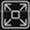, pan, zoom, and autoscalebuttons. You can also zoom and pan using your mouse.

Data Types: `char` | `string`

Specify the number of updates before scaling as a real, positive scalar integer.

Tunable: Yes

#### Dependency

To enable this property, set `AxesScaling` to `"Updates"`.

Data Types: `double`

### Measurements

Channel for which to obtain measurements, specified as a positive integer in the range [1 N], where N is the number of input channels.

Tunable: Yes

#### Scope Window Use

Click the Measurements tab on the Dynamic Filter Visualizer toolstrip. In the Channel section, select a Channel.

Data Types: `double`

Cursor measurements to display waveform cursors, specified as a `CursorMeasurementsConfiguration` object.

All `CursorMeasurementsConfiguration` properties are tunable.

Tunable: Yes

#### Scope Window Use

Click the Measurements tab on the Dynamic Filter Visualizer toolstrip and modify the cursor measurements in the Cursors section.

Peak finder measurements to compute and display the largest calculated peak values, specified as a `PeakFinderConfiguration` object.

All `PeakFinderConfiguration` properties are tunable.

Tunable: Yes

#### Scope Window Use

Click the Measurements tab on the Dynamic Filter Visualizer toolstrip and modify the peak finder measurements in the Peaks section.

### Visualization

Caption to display on the Dynamic Filter Visualizer window, specified as a character vector or a string scalar.

Example: `'Dynamic Filter Visualizer'`

Example: `"Dynamic Filter Visualizer"`

Tunable: Yes

Scope window position in pixels, specified as a four-element double vector of the form [left bottom width height]. The default value of this property is dependent on the screen resolution, and is such that the window is positioned in the center of the screen, with a width and height of `800` and `500` pixels, respectively.

Tunable: Yes

Data Types: `single` | `double` | `int8` | `int16` | `int32` | `int64` | `uint8` | `uint16` | `uint32` | `uint64`

Specify whether to display the filter visualizer in maximized-axes mode. In this mode, the axes are expanded to fit into the entire display. To conserve space, labels do not appear in each display. Instead, tick-mark values appear on top of the plotted data. You can select one of the following options:

• `"Auto"` — The axes appear maximized in all displays only if the `Title` and `YLabel` properties are empty for every display. If you enter any value in any display for either of these properties, the axes are not maximized.

• `"On"` — The axes appear maximized in all displays. Any values entered into the `Title` and `YLabel` properties are hidden.

• `"Off"` — None of the axes appear maximized.

Tunable: Yes

#### Scope Window Use

Hover over the Dynamic Filter Visualizer window to see the maximize axes button.

Data Types: `char` | `string`

Display title, specified as a character vector or a string scalar.

Example: `'Magnitude Response'`

Example: `"Magnitude Response"`

Tunable: Yes

#### Scope Window Use

In the section on the Plot tab of the Dynamic Filter Visualizer toolstrip, click . In the Filter Visualizer Settings window that opens, under Display and Labels, set Title to a character vector or a string scalar.

Y-axis limits, specified as a two-element numeric row vector with the second element greater than the first element and of the form [ymin, ymax].

Tunable: Yes

#### Scope Window Use

In the section on the Plot tab of the Dynamic Filter Visualizer toolstrip, click . In the Filter Visualizer Settings window that opens, under Data and Axes, set Y-Axis Limits to a two-element numeric vector.

Data Types: `single` | `double` | `int8` | `int16` | `int32` | `int64` | `uint8` | `uint16` | `uint32` | `uint64`

When this property is set to `false`, no legend is displayed. When this property is set to `true`, a legend with automatic string labels for each input filter is displayed.

Tunable: Yes

#### Scope Window Use

In the section on the Plot tab of the Dynamic Filter Visualizer toolstrip, click .

Data Types: `logical`

Names to label the input filters in the legend, specified as a cell array of character vectors or an array of strings. The default is an empty cell array. When this property is set to an empty cell array, the filters are named by default names, such as `Filter 1`, `Filter 2`, and so on.

Tunable: Yes

#### Scope Window Use

In the section on the Plot tab of the Dynamic Filter Visualizer toolstrip, click . In the legend that appears on the plot, click the filter name.

Set this property to `true` to show grid lines on the plot.

#### Scope Window Use

In the section on the Plot tab of the Dynamic Filter Visualizer toolstrip, click . In the Filter Visualizer Settings window that opens, under Data and Axes, select Grid.

Upper limit spectral mask, specified as a two-column matrix. The first column represents the frequency values (Hz), and the second column represents the magnitude spectrum of the upper limit mask.

Tunable: Yes

Data Types: `single` | `double` | `int8` | `int16` | `int32` | `int64` | `uint8` | `uint16` | `uint32` | `uint64`

Lower limit spectral mask, specified as a two-column matrix. The first column represents the frequency values (Hz), and the second column represents the magnitude spectrum of the lower limit mask.

Tunable: Yes

Data Types: `single` | `double` | `int8` | `int16` | `int32` | `int64` | `uint8` | `uint16` | `uint32` | `uint64`

## Usage

### Syntax

``dfv(filt)``
``dfv(B,A)``

### Description

````dfv(filt)` displays the time-varying magnitude response of the object filter, `filt`, in the Dynamic Filter Visualizer figure, as long as `filt` has a valid `freqz()` implementation.```
````dfv(B,A)` displays the magnitude response for the digital filters with numerator and denominator polynomial coefficients stored in `B1` and `A1`, `B2` and `A2`, ..., and `BN` and `AN`, respectively.```

### Input Arguments

expand all

Input filter System object with a valid `freqz()` implementation.

Numerator polynomial coefficients, specified as a row vector.

Data Types: `single` | `double`

Denominator polynomial coefficients, specified as a:

• scalar –– The filter is an FIR filter.

• row vector –– The filter is an IIR filter.

Data Types: `single` | `double`

## Object Functions

expand all

 `step` Display time-varying magnitude response `printToFigure` Print scope window to MATLAB figure
 `show` Display scope window `hide` Hide scope window `isVisible` Determine visibility of scope `generateScript` Generate MATLAB script to create scope with current settings

## Examples

collapse all

Design an FIR filter with a time-varying magnitude and phase response. Plot this varying response on a dynamic filter visualizer in normalized frequency units.

Create a `dsp.DynamicFilterVisualizer` object. Set the `PlotAsMagnitudePhase` and the `NormalizedFrequency` properties to `true`.

```dfv = dsp.DynamicFilterVisualizer(PlotAsMagnitudePhase=1,... NormalizedFrequency=true,ShowLegend=true,... Title='Magnitude and Phase Response',... FilterNames="FIR Filter")```
```dfv = dsp.DynamicFilterVisualizer handle with properties: FFTLength: 2048 NormalizedFrequency: 1 FrequencyRange: [0 1] XScale: 'Linear' MagnitudeDisplay: 'Magnitude (dB)' PlotAsMagnitudePhase: 1 PlotType: 'Line' AxesScaling: 'Auto' Show all properties ```

Vary the cutoff frequency of the FIR filter k from `0.1` to `0.5` in increments of `0.001`. View the varying magnitude and phase response using the dynamic filter visualizer.

```for k = 0.1:0.001:0.5 b = designLowpassFIR(FilterOrder=90,CutoffFrequency=k); dfv(b,1); end```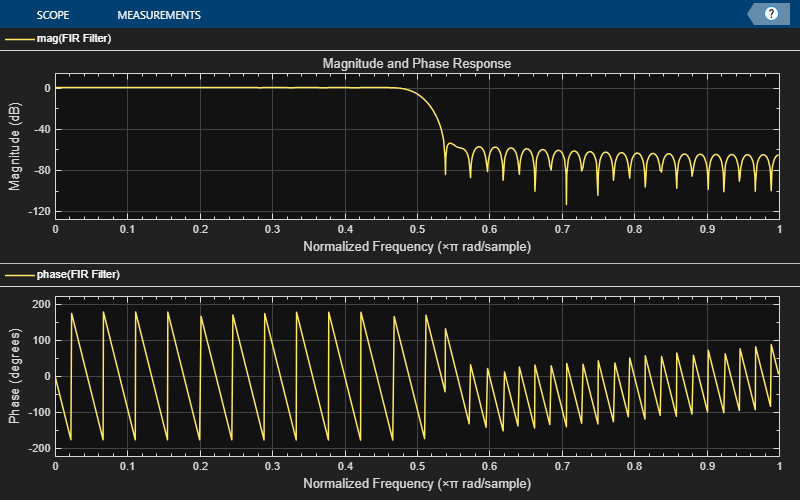Visualize the varying magnitude response of the variable bandwidth FIR filter using the dynamic filter visualizer.

Create a `dsp.DynamicFilterVisualizer` object.

```dfv = dsp.DynamicFilterVisualizer(YLimits=[-160 10],... FilterNames="Variable Bandwidth FIR Filter")```
```dfv = dsp.DynamicFilterVisualizer handle with properties: FFTLength: 2048 NormalizedFrequency: 0 SampleRate: 44100 FrequencyRange: [0 22050] XScale: 'Linear' MagnitudeDisplay: 'Magnitude (dB)' PlotAsMagnitudePhase: 0 PlotType: 'Line' AxesScaling: 'Manual' Show all properties ```

Design a bandpass variable bandwidth FIR filter with a center frequency of 5 kHz and a bandwidth of 4 kHz.

```Fs = 44100; vbw = dsp.VariableBandwidthFIRFilter(FilterType='Bandpass',... FilterOrder=100,... SampleRate=Fs,... CenterFrequency=5e3,... Bandwidth=4e3);```

Vary the center frequency of the filter. Visualize the varying magnitude response of the filter using the `dsp.DynamicFilterVisualizer` object.

```for idx = 1:100 dfv(vbw); vbw.CenterFrequency = vbw.CenterFrequency + 20; end```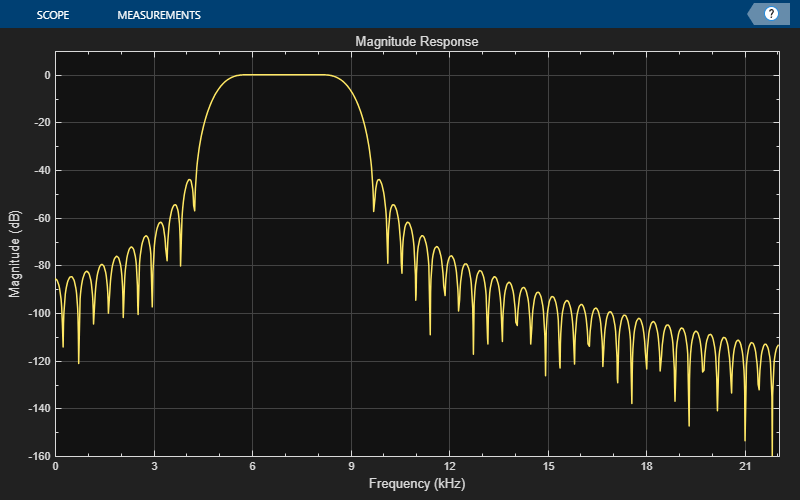Since R2023b

Use the `printToFigure` function to print the `dsp.DynamicFilterVisualizer` object display window to a new MATLAB® figure.

Create a `dsp.DynamicFilterVisualizer` object.

`dfv = dsp.DynamicFilterVisualizer(YLimits=[-120 10]);`

Design FIR filter with varying cutoff frequencies ranging from 0.1 to 0.5. Plot the magnitude response of the filter using the Dynamic Filter Visualizer.

```for k = 0.1:0.001:0.5 b = fir1(90, k); dfv(b,1); end ```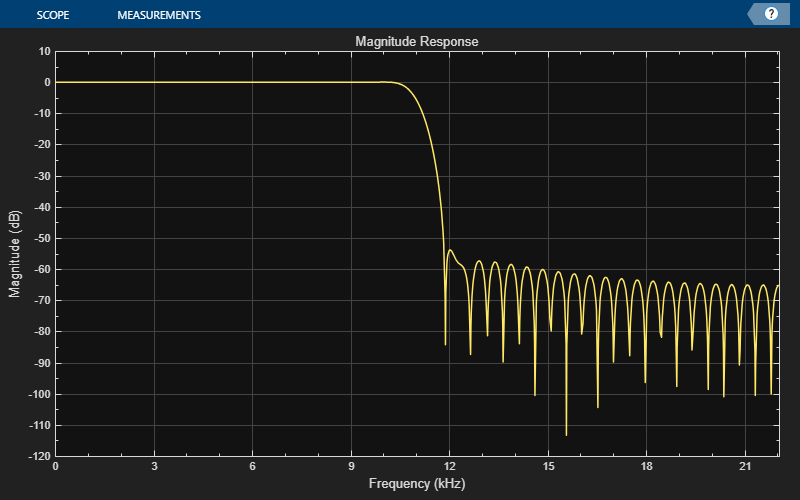Print the display of the magnitude response to a new MATLAB figure. The function returns a handle to the figure.

`scopeFig = printToFigure(dfv);`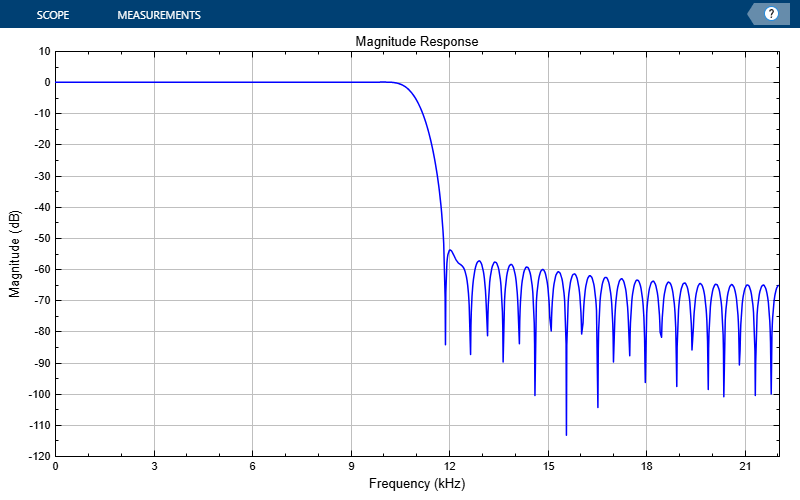The handle to the figure `scopeFig` lets you modify the appearance and the behavior of the figure window.

Specify a figure name and change the size of the figure to 400-by-250 pixels.

```scopeFig.Name="Magnitude Response of FIR Filter"; scopeFig.NumberTitle="off"; scopeFig.Position=[1 1 400 250];```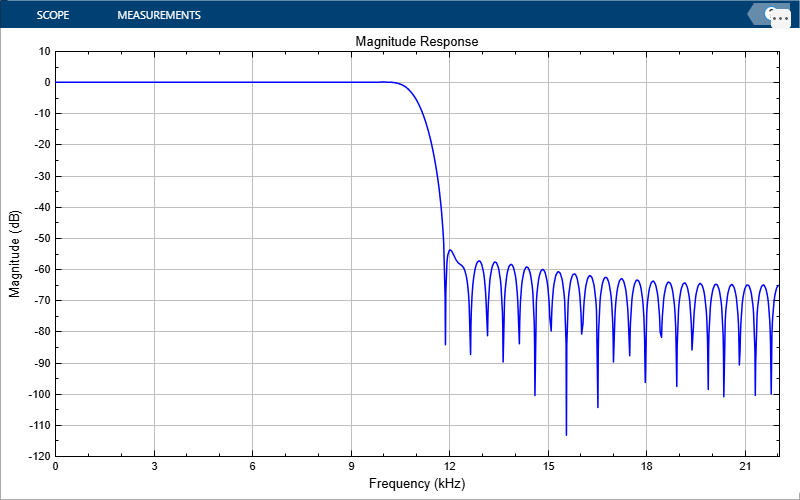When printing to figure, you can make the figure invisible by setting the `Visible` argument to `false`.

`scopeFig = printToFigure(dfv,Visible=false);`

## Limitations

• Does not support C/C++ code generation using MATLAB® Coder™. To generate a standalone application, use the MATLAB Compiler™.

• Supports MEX code generation by treating the calls to the object as extrinsic.

## Version History

Introduced in R2018b

expand all